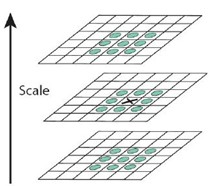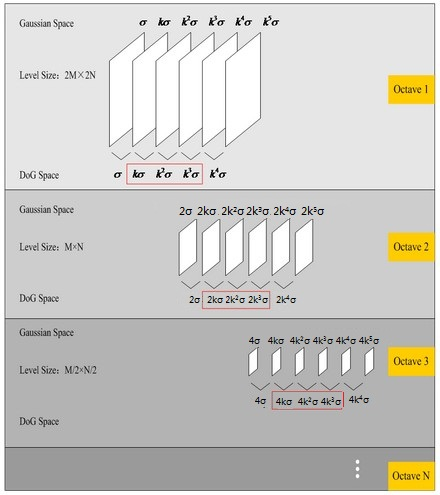# SIFT特征详解

## 1.SIFT概述

SIFT的全称是Scale Invariant Feature Transform，尺度不变特征变换，由加拿大教授David G.Lowe提出的。SIFT特征对旋转、尺度缩放、亮度变化等保持不变性，是一种非常稳定的局部特征。

### 1.1 SIFT算法具的特点

1. 图像的局部特征，对旋转、尺度缩放、亮度变化保持不变，对视角变化、仿射变换、噪声也保持一定程度的稳定性。
2. 独特性好，信息量丰富，适用于海量特征库进行快速、准确的匹配。
3. 多量性，即使是很少几个物体也可以产生大量的SIFT特征
4. 高速性，经优化的SIFT匹配算法甚至可以达到实时性
5. 扩招性，可以很方便的与其他的特征向量进行联合。

### 1.2 SIFT特征检测的步骤

1. 尺度空间的极值检测 搜索所有尺度空间上的图像，通过高斯微分函数来识别潜在的对尺度和选择不变的兴趣点。
2. 特征点定位 在每个候选的位置上，通过一个拟合精细模型来确定位置尺度，关键点的选取依据他们的稳定程度。
3. 特征方向赋值 基于图像局部的梯度方向，分配给每个关键点位置一个或多个方向，后续的所有操作都是对于关键点的方向、尺度和位置进行变换，从而提供这些特征的不变性。
4. 特征点描述 在每个特征点周围的邻域内，在选定的尺度上测量图像的局部梯度，这些梯度被变换成一种表示，这种表示允许比较大的局部形状的变形和光照变换。

## 2. 尺度空间

#### 2.1 多分辨率图像金字塔

1. 对原始图像进行平滑
2. 对处理后的图像进行降采样（通常是水平、垂直方向的1/2）
降采样后得到一系列不断尺寸缩小的图像。显然，一个传统的金字塔中，每一层的图像是其上一层图像长、高的各一半。多分辨率的图像金字塔虽然生成简单，但其本质是降采样，图像的局部特征则难以保持，也就是无法保持特征的尺度不变性。

#### 2.2 高斯尺度空间

$L(x,y,\sigma) = G(x,y,\sigma) * I(x,y)$

$G(x,y,\sigma) = \frac{1}{2 \pi \sigma ^2} e ^ {\frac{x^2 +y^2}{2 \sigma^2}}$

$$\sigma$$称为尺度空间因子，它是高斯正态分布的标准差，反映了图像被模糊的程度，其值越大图像越模糊，对应的尺度也就越大。$$L(x,y,\sigma)$$代表着图像的高斯尺度空间。

$\Delta ^2 = \frac{\partial ^2}{\partial x^2} + \frac{\partial ^2}{\partial y^2}$

使用LoG虽然能较好的检测到图像中的特征点，但是其运算量过大，通常可使用**DoG（差分高斯，Difference of Gaussina）**来近似计算LoG[Marr and Hidreth]。


$$k$$为相邻两个高斯尺度空间的比例因子，则DoG的定义：

$D(x,y,\sigma) = [G(x,y,k\sigma) - G(x,y,\sigma)] \ast I(x,y) \\ = L(x,y,k\sigma) - L(x,y,\sigma)$

$o = [\log _2min(m,n)] - a$

$$o$$表示高斯金字塔的层数，m，n分别是图像的行和列。减去的系数$$a$$可以在$$0-\log_2min(m,n)$$之间的任意值，和具体需要的金字塔的顶层图像的大小有关。

$\sigma(o,s) = \sigma_0 \cdot 2^{\frac{o + s}{S}}$

$\sigma_{s+1}=k\cdot\sigma_s=2^{\frac{1}{S}}\cdot\sigma_s$

$\sigma_{o+1} = 2\sigma_o$

### 2.3 高斯金字塔构建示例

1. 金字塔的组数，$$\log_2512 = 9$$，减去因子3，构建的金字塔的组数为6。取每组的层数为3。
2. 构建第0组，将图像的宽和高都增加一倍，变成$$1024 \times 1024$$$$I_0$$）。第0层$$I_0 \ast G(x,y,\sigma_0)$$，第1层$$I_0 \ast G(x,y,k\sigma_0)$$，第2层$$I_0 \ast G(x,y,k^2\sigma_0)$$
3. 构建第1组，对$$I_0$$降采样变成$$512 \times 512$$$$I_1$$）。第0层$$I_1 \ast G(x,y,2\sigma_0)$$，第1层$$I_1 \ast G(x,y,2k\sigma_0)$$$$I_1 \ast G(x,y,2k^2\sigma_0)$$
4. $$\vdots$$
5. 构建第o组，第s层 $$I_o \ast G(x,y,2^ok^s\sigma_0)$$

## 3. DoG空间极值检测### 3.1 尺度变化的连续性

$$S=3$$，也就是每组有3层，则$$k = 2 ^ {\frac{1}{S}} = 2 ^ {\frac{1}{3}}$$，也就是有高斯金字塔每组有$$(S-1)3层图像，DoG金字塔每组有$$(S-2)2层图像。在DoG金字塔的第一组有两层尺度分别是$$\sigma,k\sigma$$，第二组有两层的尺度分别是$$2\sigma,2k\sigma$$，由于只有两项是无法比较取得极值的（只有左右两边都有值才能有极值）。由于无法比较取得极值，那么我们就需要继续对每组的图像进行高斯模糊，使得尺度形成$$\sigma,k\sigma,k^2\sigma,k^3\sigma,k^4\sigma$$，这样就可以选择中间的三项$$k\sigma,k^2\sigma,k^3\sigma$$。对应的下一组由上一组降采样得到的三项是$$2k\sigma,2k^2\sigma,2k^3\sigma$$，其首项$$2k\sigma = 2\cdot2^{\frac{1}{3}}\sigma=2^{\frac{4}{3}}\sigma$$，刚好与上一组的最后一项$$k^3\sigma = 2^{\frac{3}{3}}\sigma$$的尺度连续起来。## 4. 删除不好的极值点（特征点）

1. 低对比度的特征点
2. 不稳定的边缘响应点

### 4.1 剔除低对比度的特征点

$D(x) = D + \frac{\partial D^T}{\partial x}\Delta x+ \frac{1}{2}\Delta x^T\frac{\partial ^2D}{\partial x^2}\Delta x$

$\Delta x = -\frac{\partial^2D^{-1}}{\partial x^2}\frac{\partial D(x)}{\partial x}$

$D(\hat{x}) = D +\frac{1}{2}\frac{\partial D^T}{\partial x}\hat{x}$

### 4.2 剔除不稳定的边缘响应点

$H = \left[ \begin{array}{cc} D_{xx} \quad D_{yx} \\ D_{xy} \quad D_{yy} \end{array} \right]$

$Tr(H) = D_{xx} + D_{yy} = \alpha +\beta \\ Det(H) = D_{xx} + D_{yy} - D_{xy}^2 = \alpha \cdot \beta$

$$\gamma = \frac{\alpha}{\beta}$$ 表示最大特征值和最小特征值的比值，则

$\frac{Tr(H)^2}{Det(H)} = \frac{(\alpha +\beta)^2}{\alpha \beta} = \frac{(\gamma \beta + \beta)^2}{\gamma \beta ^2} = \frac{(\gamma +1)^2}{\gamma}$

$\frac{Tr(H)^2}{Det(H)} > \frac{(T_{\gamma} + 1)^2}{T_{\gamma}}$

## 5. 求取特征点的主方向

$L(x,y) = G(x,y,\sigma) \ast I(x,y)$

$m(x,y) = \sqrt{[L(x+1,y) - L(x-1,y)]^2 + [L(x,y + 1) - L(x,y - 1)]^2} \\$

$\theta(x,y) = \arctan{\frac{L(x,y+1) - L(x,y - 1)}{L(x + 1,y) - L(x-1,y)}}$

15%的关键点具有多方向，而且这些点对匹配的稳定性很关键。

## 6. 生成特征描述

1. 校正旋转主方向，确保旋转不变性。
2. 生成描述子，最终形成一个128维的特征向量
3. 归一化处理，将特征向量长度进行归一化处理，进一步去除光照的影响。

$\left[ \begin{array}{c} x ^ \prime \\ y ^ \prime \end{array} \right] = \left[ \begin{array}{cc} \cos \theta \quad - \sin \theta \\ \sin \theta \quad \cos \theta \end{array} \right] \left[ \begin{array}{c} x \\ y \end{array}\right]$## 7. 总结

SIFT特征以其对旋转、尺度缩放、亮度等保持不变性，是一种非常稳定的局部特征，在图像处理和计算机视觉领域有着很重要的作用，其本身也是非常复杂的，下面对其计算过程做一个粗略总结。

1. DoG尺度空间的极值检测。 首先是构造DoG尺度空间，在SIFT中使用不同参数的高斯模糊来表示不同的尺度空间。而构造尺度空间是为了检测在不同尺度下都存在的特征点，特征点的检测比较常用的方法是$$\Delta ^2G$$（高斯拉普拉斯LoG），但是LoG的运算量是比较大的，Marr和Hidreth曾指出，可以使用DoG（差分高斯）来近似计算LoG，所以在DoG的尺度空间下检测极值点。
2. 删除不稳定的极值点。主要删除两类：低对比度的极值点以及不稳定的边缘响应点。
3. ** 确定特征点的主方向**。以特征点的为中心、以$$3 \times 1.5\sigma$$为半径的领域内计算各个像素点的梯度的幅角和幅值，然后使用直方图对梯度的幅角进行统计。直方图的横轴是梯度的方向，纵轴为梯度方向对应梯度幅值的累加值，直方图中最高峰所对应的方向即为特征点的方向。
4. 生成特征点的描述子。 首先将坐标轴旋转为特征点的方向，以特征点为中心的$$16 \times 16$$的窗口的像素的梯度幅值和方向，将窗口内的像素分成16块，每块是其像素内8个方向的直方图统计，共可形成128维的特征向量。
posted @ 2015-10-03 12:33  Brook_icv  阅读(128710)  评论(13编辑  收藏  举报